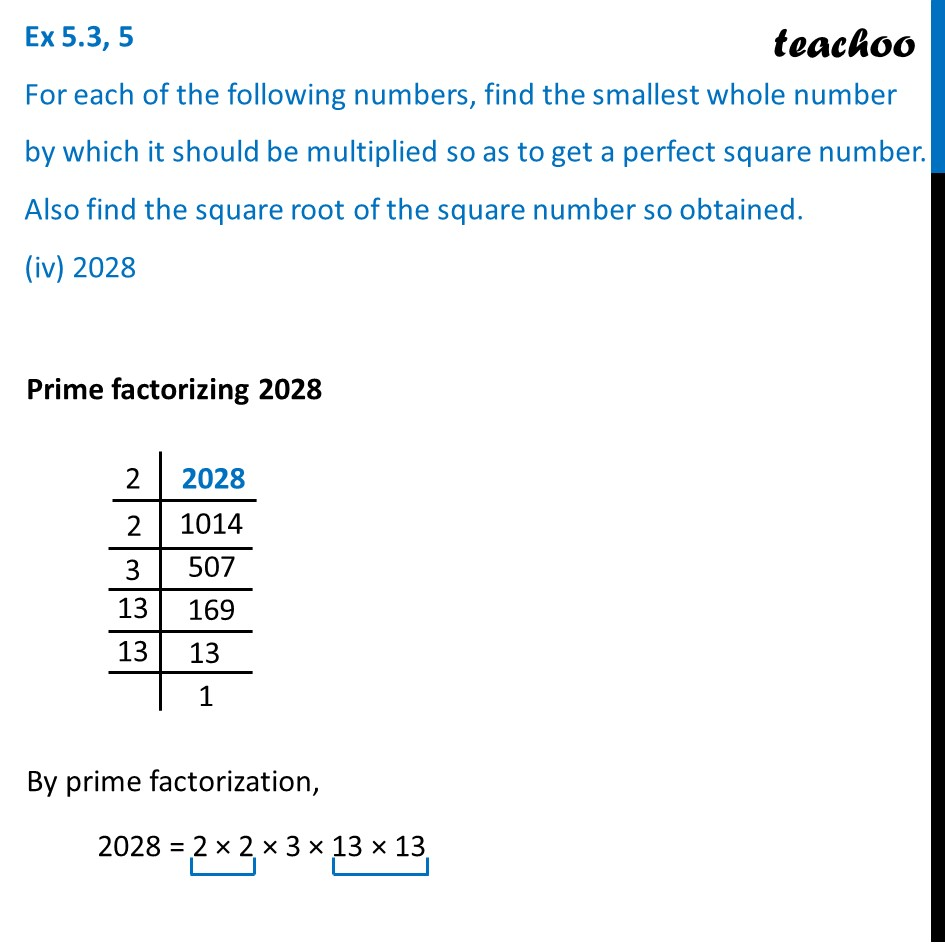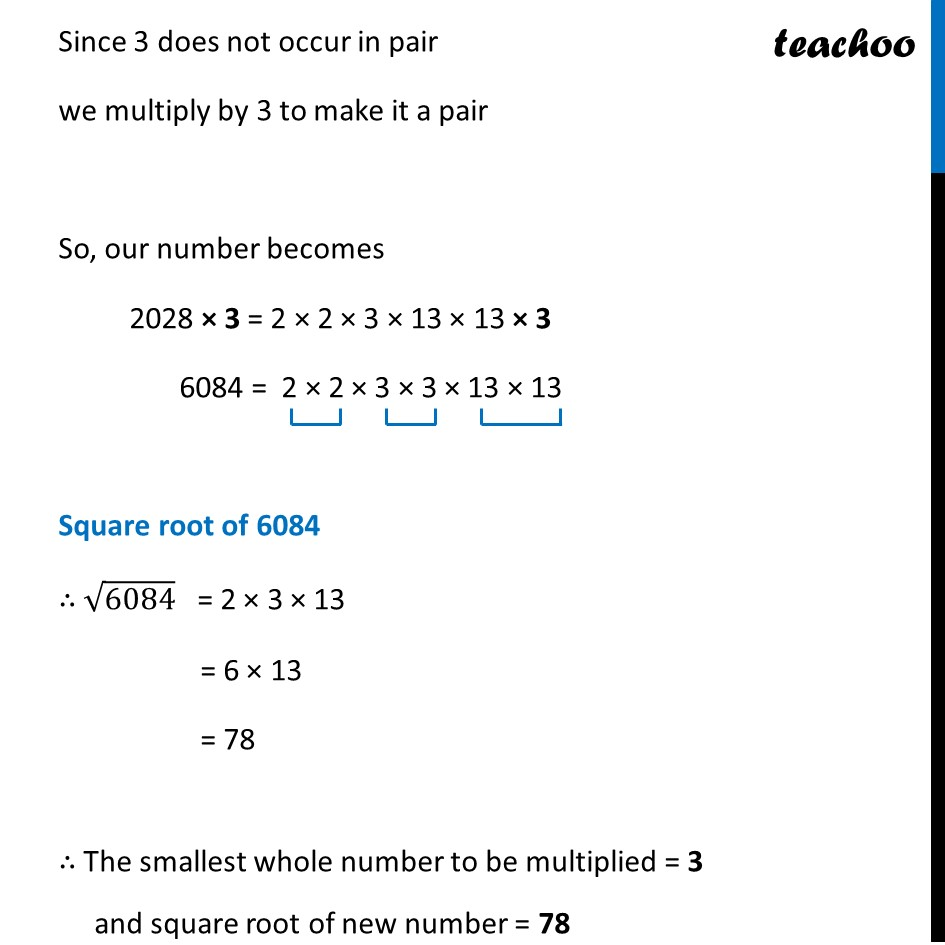Ex 5.3

Chapter 5 Class 8 Squares and Square Roots
Serial order wiseLearn in your speed, with individual attention - Teachoo Maths 1-on-1 Class

### Transcript

Ex 5.3, 5 For each of the following numbers, find the smallest whole number by which it should be multiplied so as to get a perfect square number. Also find the square root of the square number so obtained. (iv) 2028 Prime factorizing 2028 By prime factorization, 2028 = 2 × 2 × 3 × 13 × 13 Since 3 does not occur in pair we multiply by 3 to make it a pair So, our number becomes 2028 × 3 = 2 × 2 × 3 × 13 × 13 × 3 6084 = 2 × 2 × 3 × 3 × 13 × 13 Square root of 6084 ∴ √6084 = 2 × 3 × 13 = 6 × 13 = 78 ∴ The smallest whole number to be multiplied = 3 and square root of new number = 78